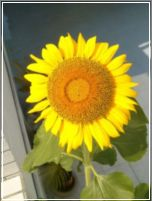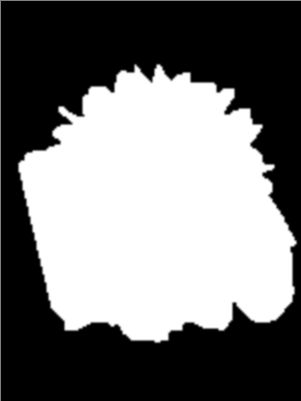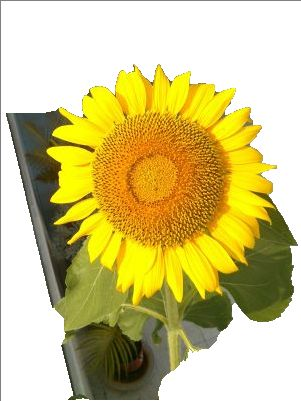## Thursday, August 08, 2013

### Downsampling images to speed up GrabCut

In the previous post  I've tried the GrabCut function of OpenCV, when I noticed it was time consuming but didn't try to check the exact processing time. But I was still wondering ``how slow'' did GrabCut could be. So, I started to add the clock() function to see the result .

Later, I though of that the processing time was greatly affected by the image size, so I searched for functions which could reduce the images for speeding up GrabCut. What I found were cv::pyrDown() and cv::pyrUp() and they've been implemented in my test code (listed below).

```#include "opencv2/opencv.hpp"
#include <iostream>
#include <time.h>

using namespace std;

const bool DOWN_SAMPLED = true;
const unsigned int BORDER = 1;
const unsigned int BORDER2 = BORDER + BORDER;

int main( )
{
clock_t tStart_all = clock();
// Open another image
cv::Mat image;

if(! image.data ) // Check for invalid input
{
cout <<  "Could not open or find the image" << std::endl ;
return -1;
}

cv::Mat result; // segmentation result (4 possible values)
cv::Mat bgModel,fgModel; // the models (internally used)

if(DOWN_SAMPLED){
// downsample the image
cv::Mat downsampled;
cv::pyrDown(image, downsampled, cv::Size(image.cols/2, image.rows/2));

cv::Rect rectangle(BORDER,BORDER,downsampled.cols-BORDER2,downsampled.rows-BORDER2);

clock_t tStart = clock();
// GrabCut segmentation
cv::grabCut(downsampled,    // input image
result,   // segmentation result
rectangle,// rectangle containing foreground
bgModel,fgModel, // models
1,        // number of iterations
cv::GC_INIT_WITH_RECT); // use rectangle
printf("Time taken by GrabCut with downsampled image: %f s\n", (clock() - tStart)/(double)CLOCKS_PER_SEC);

// Get the pixels marked as likely foreground
cv::compare(result,cv::GC_PR_FGD,result,cv::CMP_EQ);
cv::Mat resultUp;
cv::pyrUp(result, resultUp, cv::Size(result.cols*2, result.rows*2));
// Generate output image
cv::Mat foreground(image.size(),CV_8UC3,cv::Scalar(255,255,255));
image.copyTo(foreground,resultUp); // bg pixels not copied

// display original image
cv::namedWindow("Image");
cv::imshow("Image",image);

// display downsampled image
cv::rectangle(downsampled, rectangle, cv::Scalar(255,255,255),1);
cv::namedWindow("Downsampled Image");
cv::imshow("Downsampled Image",downsampled);

// display result
cv::namedWindow("Segmented Image");
cv::imshow("Segmented Image",foreground);
}
else {
cv::Rect rectangle(BORDER,BORDER,image.cols-BORDER2,image.rows-BORDER2);

clock_t tStart = clock();
// GrabCut segmentation
cv::grabCut(image,    // input image
result,   // segmentation result
rectangle,// rectangle containing foreground
bgModel,fgModel, // models
1,        // number of iterations
cv::GC_INIT_WITH_RECT); // use rectangle
printf("Time taken by GrabCut with original image: %f s\n", (clock() - tStart)/(double)CLOCKS_PER_SEC);

// Get the pixels marked as likely foreground
cv::compare(result,cv::GC_PR_FGD,result,cv::CMP_EQ);
// Generate output image
cv::Mat foreground(image.size(),CV_8UC3,cv::Scalar(255,255,255));
image.copyTo(foreground,result); // bg pixels not copied

// display original image
cv::rectangle(image, rectangle, cv::Scalar(255,255,255),1);
cv::namedWindow("Image");
cv::imshow("Image",image);

// display result
cv::namedWindow("Segmented Image");
cv::imshow("Segmented Image",foreground);
}

printf("Total processing time: %f s\n", (clock() - tStart_all)/(double)CLOCKS_PER_SEC);

cv::waitKey();
return 0;
}
```

The key idea was to downsample the image for GrabCut and then upsample the result (I thought it was a mask) to the original size. The result showed a remarkable speeding up in both the debug and the release mode.

Here are the output images with the downsampling strategy:Fig 1. Original imageFig 2. Downsampled imageFig 3. Mask obtained by using GrabCutFig 4. Upsampled maskFig 5. Final result
Here is the result without the downsampling strategy:Fig 6. GrabCut result without downsampling

Comparing Figure 5 and 6, we can easily notice the differences between the segmented results. When applying the downsampling strategy, some image details were lost and the mask would be different and had rougher edges as well.

Although the downsampling strategy has the drawback of losing image details, the benefit of reducing processing time was significant. The following table lists the processing time obtained by using above code with and without the downsampling strategy.

processing time (sec.)without downsamplingwith downsampling
debug modeGrabCut3.0780.717
Total3.1010.756
release modeGrabCut0.5990.123
Total0.6190.157

---
 Try GrabCut using OpenCV
 How to use clock() in C++